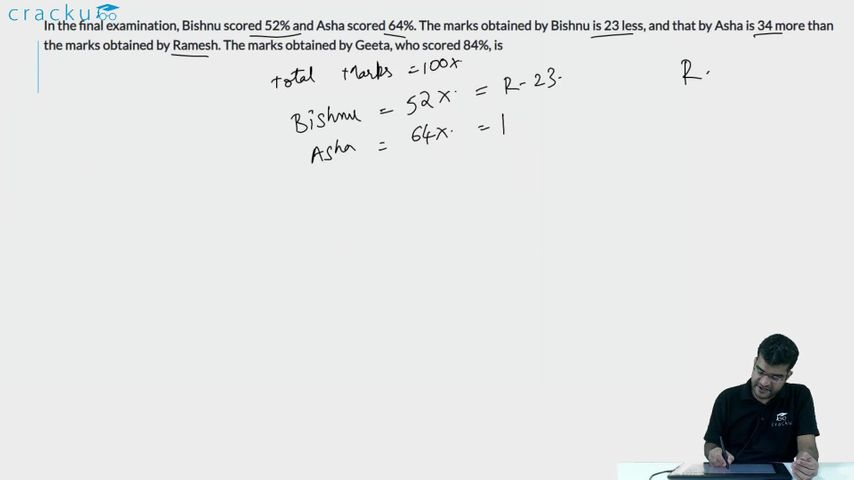Question 61

# In the final examination, Bishnu scored 52% and Asha scored 64%. The marks obtained by Bishnu is 23 less, and that by Asha is 34 more than the marks obtained by Ramesh. The marks obtained by Geeta, who scored 84%, is

Solution

Let the total marks be 100x

Marks obtained by Bishnu = 52x

Marks obtained by Asha = 64x

Marks obtained by Ramesh = 52x+23

Marks obtained by Ramesh = 64x-34

=> 52x+23 = 64x-34

=> x = $$\frac{19}{4}$$

Marks obtained by Geeta =84x = 84*19/4 = 399

### View Video Solution